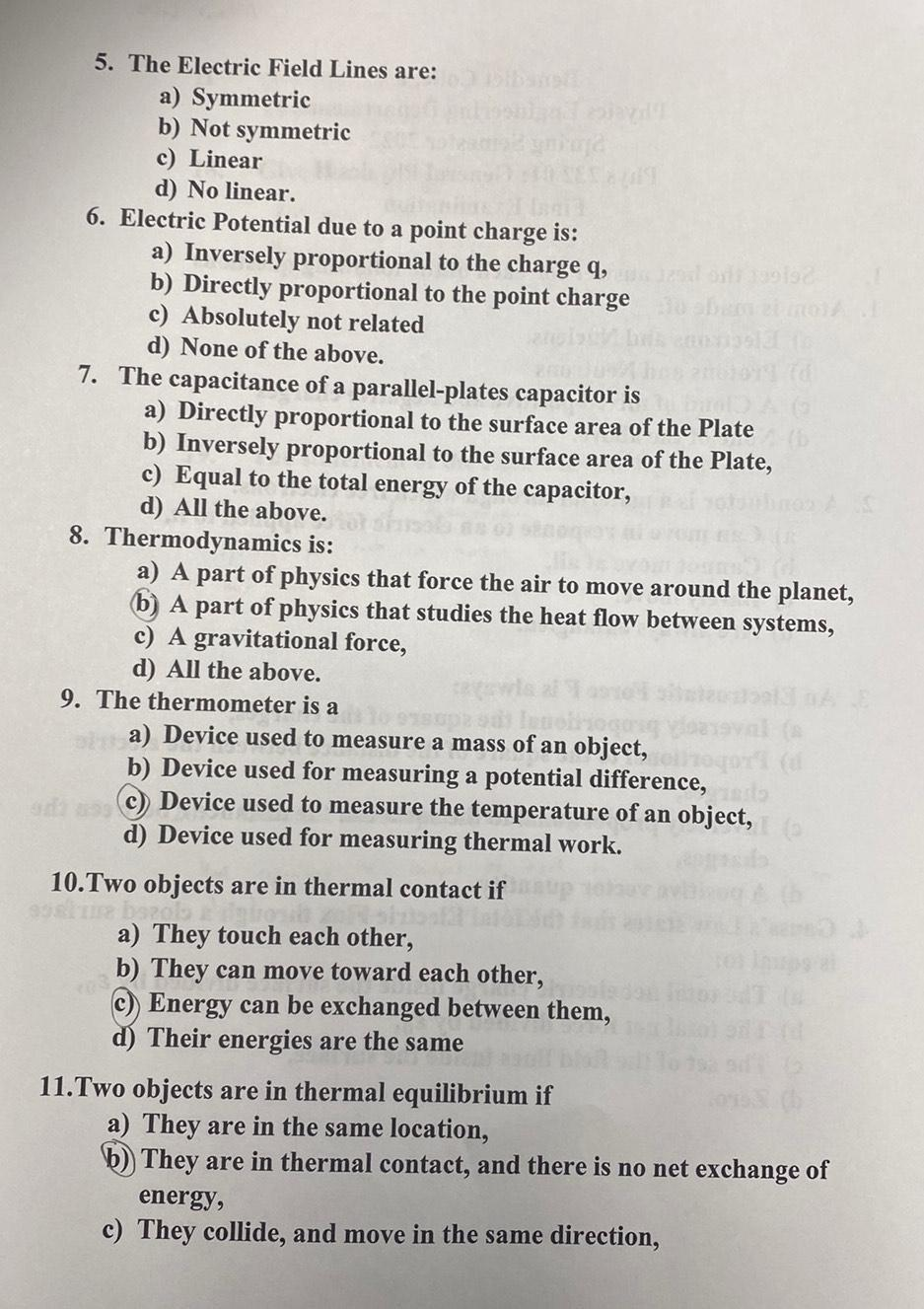Question:

# 5. The Electric Field Lines are: a) Symmetric b) Not symmetric c) Linear d) No linear. 6. Electric Potential due to a point char5. The Electric Field Lines are: a) Symmetric b) Not symmetric c) Linear d) No linear. 6. Electric Potential due to a point charge is: a) Inversely proportional to the charge q, 1998 b) Directly proportional to the point charge c) Absolutely not related d) None of the above. 7. The capacitance of a parallel-plates capacitor is a) Directly proportional to the surface area of the Plate b) Inversely proportional to the surface area of the Plate, c) Equal to the total energy of the capacitor, d) All the above. 8. Thermodynamics is: a) A part of physics that force the air to move around the planet, b) A part of physics that studies the heat flow between systems, c) A gravitational force, d) All the above. 9. The thermometer is a pizo mo a) Device used to measure a mass of an object, b) Device used for measuring a potential difference, C) Device used to measure the temperature of an object, d) Device used for measuring thermal work. 10.Two objects are in thermal contact if a) They touch each other, b) They can move toward each other, C) Energy can be exchanged between them, d) Their energies are the same 11.Two objects are in thermal equilibrium if a) They are in the same location, b) They are in thermal contact, and there is no net exchange of energy, c) They collide, and move in the same direction,